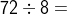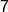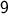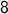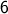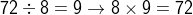# SSAT Elementary Level Math : How to divide

## Example Questions

← Previous 1 3 4 5 6 7 8 9 55 56

### Example Question #1 : How To Divide

Find the quotient.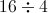Explanation:

We want to know how many groups of 4 there are in 16?

Draw groups of 4 *'s until you get to 16 *'s:

****   ****   ****   ****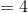groups

or

Add 4's until you get to 16: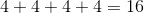(there are four 4's in 16)

### Example Question #1 : How To Divide

Four girls want to play marbles; one of them brought a bag that contains 72 marbles. If each girl is to start out with the same number of marbles, how many will each one get?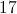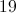Explanation:

72 marbles are being divided among the four children, so divide 72 by 4: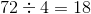### Example Question #3 : How To Divide

Ben has ten chores to do each week. If he does two chores per day, how many days each week will he have no chores to do?Explanation:

This is a two-step question. First, divide 10 chores by 2 (because he will do 2 per day) to find out how many days he will do chores.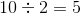He will have 5 days of chores.

Since there are 7 days in a week, subtract the number of chore days from the number of days in a week.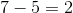He will have 2 non-chore days.

### Example Question #4 : How To Divide

Find the quotient and show a remainder if necessary.  Remainders are expressed as "R".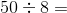6 R2

2 R6

5 R8

6 R8

6 R2

Explanation:

When you divide 8 into 50, it does not go in evenly.

8 goes into 50, 6 times with a remainder of 2.

You can also draw a picture:

oooooooo   oooooooo   oooooooo   oooooooo   oooooooo   oooooooo  oo

This shows 6 groups of 8 (equals 48) with 2 left over (remainder).

Therefore, the correct answer is 6 R2.

### Example Question #5 : How To Divide

Find the quotient, show a remainder if necessary.  Remainders are expressed as "R"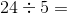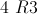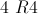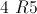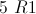Explanation:

When you divide 5 into 24, it does not go in evenly.

5 goes into 24 four times, with a remainder of 4.

You can also draw a picture:

ooooo   ooooo   ooooo   ooooo   oooo

This shows 4 groups of 5 (equals 20) with 4 left over (remainder).

Therefore, the correct answer is 4 R4.

### Example Question #6 : How To Divide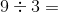Explanation: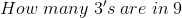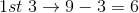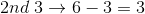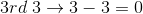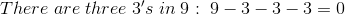### Example Question #7 : How To Divide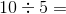Explanation: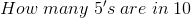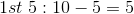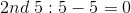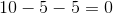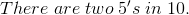### Example Question #8 : How To Divide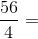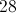Explanation: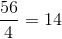### Example Question #9 : How To Divide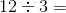Explanation:

This question is asking for the quotient when you divide 3 into 12.  Another way to think about this is how many times does 3 go into 12.

3, 6, 9, 12 so it goes in four times.

### Example Question #10 : How To Divide